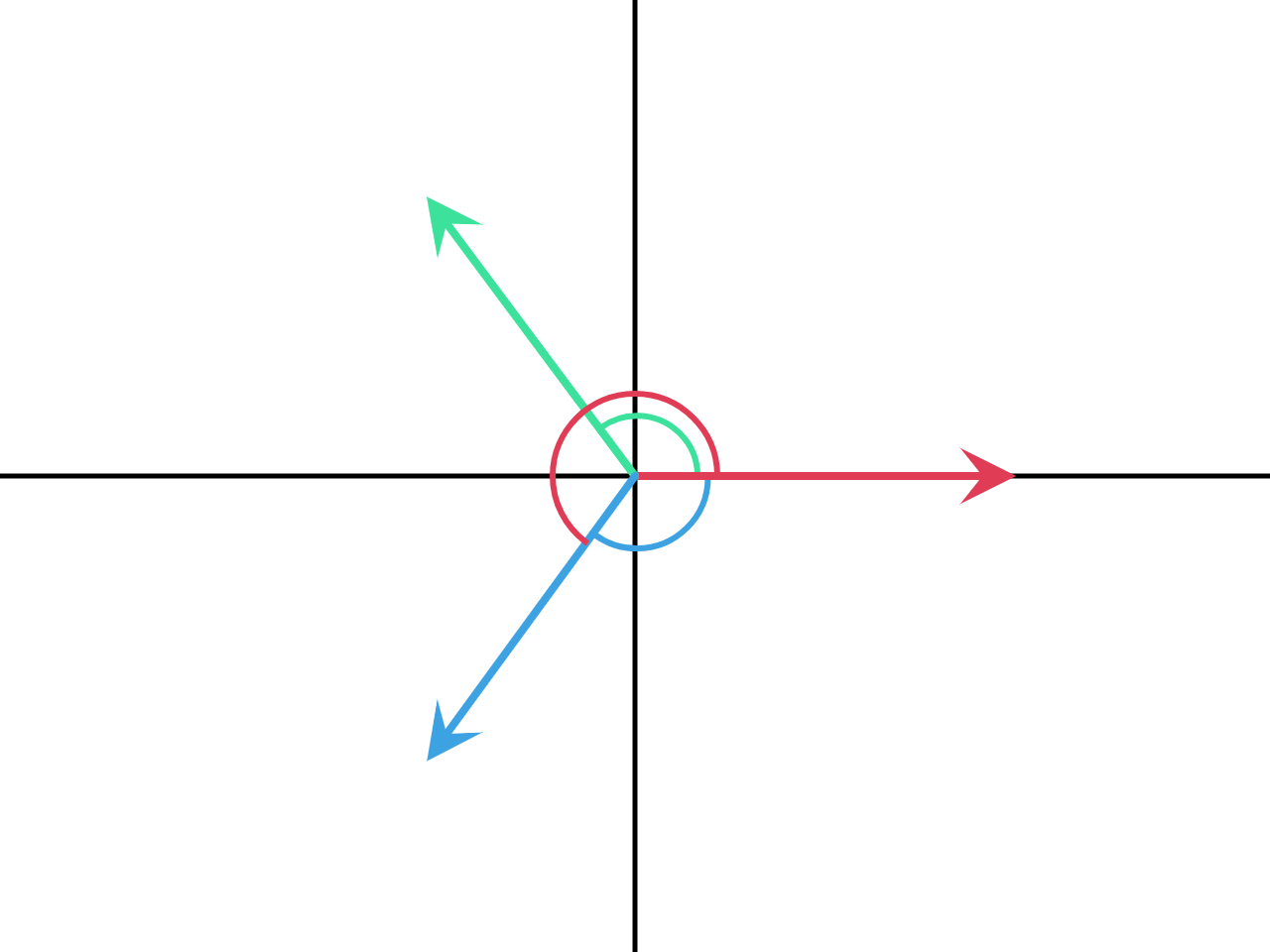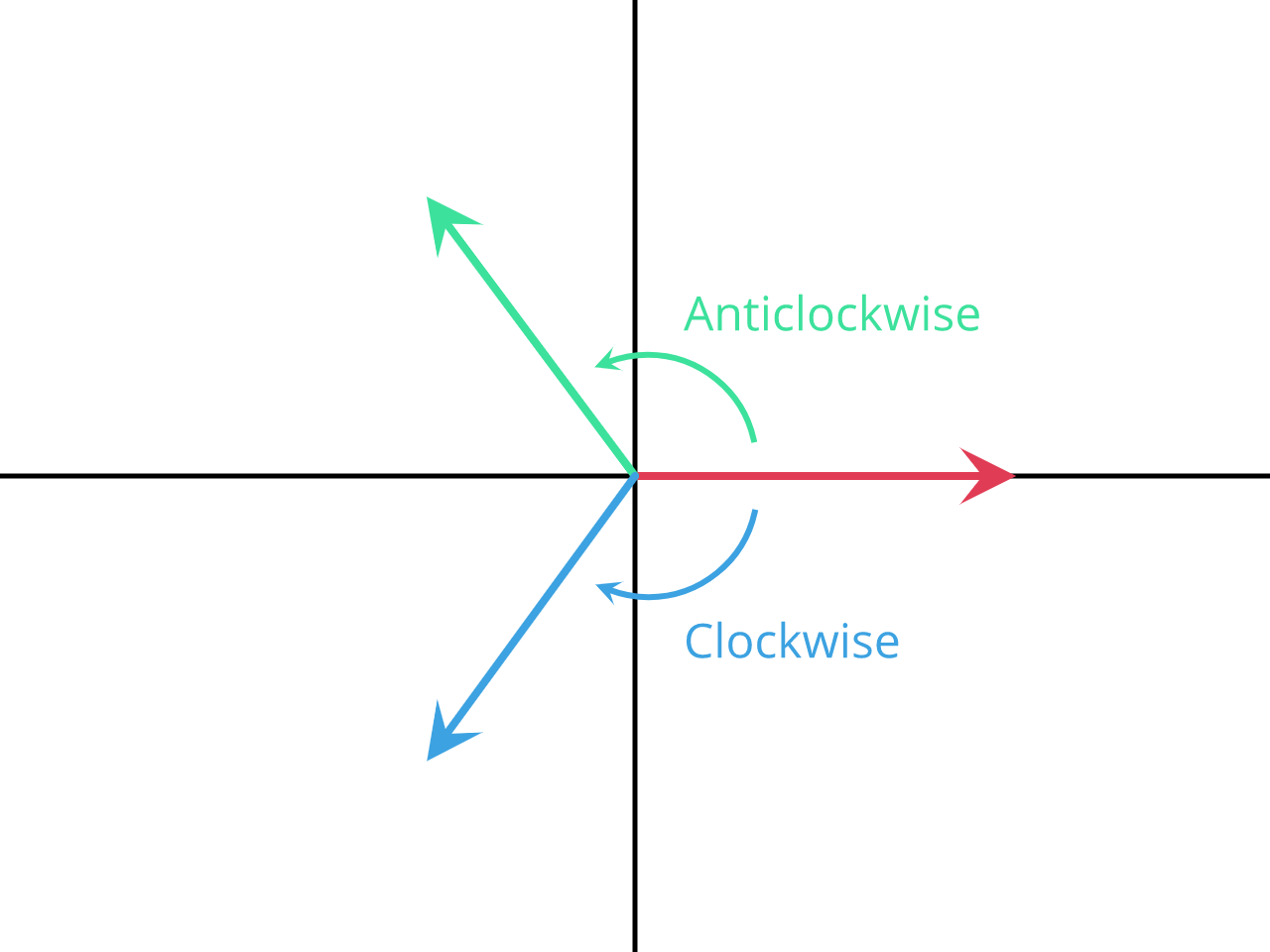# Vector Angle

## Description

This node takes two vectors and computes two things:

1. The shortest angle between the two vectors in radian.
2. The directed angle between the two vectors in a form of rotation quaternion.

## Inputs

• Vector A - The first vector.
• Vector B - The second vector.

## Outputs

• Angle - The shortest angle between the two vectors in radian.
• Rotation Difference - A quaternion that represents the directed angle between the two vectors.

## Demonstration

### Angle

The Angle output returns the shortest angle between the input vectors. This means it can not compute the angle in full `[0, 2pi]` (`[0°, 360°]`) range but rather in `[0, pi]` (`[0°, 180°]`) range, this can be demonstrated in the following illustration:The standard angle between the red and green vectors is `120°` (Green angle) while the standard angle between the red and blue vectors is `240°` (Red Angle). However, the Angle output will return `120°` in both cases, why? because there is a shorter angle in the second case which measures `120°` (Blue angle). This output doesn’t tell us about the axis of rotation as well. So we see that the angle output doesn’t provide full information about the angle.

### Rotation Difference

This output returns the directed angle between the input vectors in a form of a quaternion. A directed angle is a signed shortest angle at which the first vector has to be rotated to coincide with the second vector. To understand this, let us look at the previouse example again:The directed angle between the red and green vectors is equal to `+120°` because to rotate the red vector to coincide with the green vector along the shortest path, we rotate in the anticlockwise direction. On the other hand, the directed angle between the red and blue vectors is equal to `-120°` because to rotate the red vector to coincide with the blue vector along the shortest path, we rotate in the clockwise direction, an equivalence angle to `-120°` is `-120°+360° = 240°` which is the angle we originally computed. It should be noted that if we switched the order of the vectors, we will get negative the original directed angle; because the rotation direction changes from anticlockwise to clockwise—or the converse. So we see that the Rotation Difference output gives full information about the angles, including the axis of rotation and the direction of the rotation.